### PROC PHREG Statement

PROC PHREG <options> ;

The PROC PHREG statement invokes the PHREG procedure. Table 71.1 summarizes the options available in the PROC PHREG statement.

Table 71.1: PROC PHREG Statement Options

Option

Description

Specifies the level of significance

Displays a table that contains the number of units and the corresponding number of events in the risk sets

Uses the model-based covariance matrix in the analysis

Adds the estimated covariance matrix to the OUTEST= data set

Requests the robust sandwich estimate for the covariance matrix

Names the SAS data set to be analyzed

Requests the Schemper-Henderson predictive measures

Names the SAS data set that contains initial estimates

Recompiles the risk sets

Specifies the length of effect names

Suppresses all displayed output

Suppresses the summary display observation frequencies

Creates an output SAS data set containing estimates of the regression coefficients

Controls the plots that are produced through ODS Graphics

Displays simple descriptive statistics

Requests diagnostics based on weighted residuals for checking the proportional hazards assumption

You can specify the following options in the PROC PHREG statement.

ALPHA=number

specifies the level of significancefor% confidence intervals. The value number must be between 0 and 1; the default value is 0.05, which results in 95% intervals. This value is used as the default confidence level for limits computed by the BASELINE, BAYES, CONTRAST, HAZARDRATIO, and MODEL statements. You can override this default by specifying the ALPHA= option in the separate statements.

ATRISK

displays a table that contains the number of units at risk at each event time and the corresponding number of events in the risk sets. For example, the following risk set information is displayed if the ATRISK option is specified in the example in the section Getting Started: PHREG Procedure.

 Risk Set Information Number of Units Days At Risk Event 142 40 1 143 39 1 156 38 1296 5 2 304 3 1 323 2 1

COVOUT

adds the estimated covariance matrix of the parameter estimates to the OUTEST= data set. The COVOUT option has no effect unless the OUTEST= option is specified.

COVM

requests that the model-based covariance matrix (which is the inverse of the observed information matrix) be used in the analysis if the COVS option is also specified. The COVM option has no effect if the COVS option is not specified.

COVSANDWICH <(AGGREGATE)>
COVS <(AGGREGATE)>

requests the robust sandwich estimate of Lin and Wei (1989) for the covariance matrix. When this option is specified, this robust sandwich estimate is used in the Wald tests for testing the global null hypothesis, null hypotheses of individual parameters, and the hypotheses in the CONTRAST and TEST statements. In addition, a modified score test is computed in the testing of the global null hypothesis, and the parameter estimates table has an additional StdErrRatio column, which contains the ratios of the robust estimate of the standard error relative to the corresponding model-based estimate. Optionally, you can specify the keyword AGGREGATE enclosed in parentheses after the COVSANDWICH (or COVS) option, which requests a summing up of the score residuals for each distinct ID pattern in the computation of the robust sandwich covariance estimate. This AGGREGATE option has no effect if the ID statement is not specified.

DATA=SAS-data-set

names the SAS data set that contains the data to be analyzed. If you omit the DATA= option, the procedure uses the most recently created SAS data set.

EV

requests the Schemper-Henderson measure (Schemper and Henderson, 2000) of the proportion of variation that is explained by a Cox regression. This measure of explained variation (EV) is the ratio of distance measures between the 1/0 survival processes and the fitted survival curves with and without covariates information. The distance measure is referred to as the predictive inaccuracy, because the smaller the predictive inaccuracy, the better the prediction. When you specify this option, PROC PHREG creates a table that has three columns: one presents the predictive inaccuracy without covariates (D); one presents the predictive inaccuracy with covariates (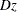); and one presents the EV measure, computed according to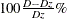.

INEST=SAS-data-set

names the SAS data set that contains initial estimates for all the parameters in the model. BY-group processing is allowed in setting up the INEST= data set. See the section INEST= Input Data Set for more information.

MULTIPASS

requests that, for each Newton-Raphson iteration, PROC PHREG recompile the risk sets corresponding to the event times for the (start,stop) style of response and recomputes the values of the time-dependent variables defined by the programming statements for each observation in the risk sets. If the MULTIPASS option is not specified, PROC PHREG computes all risk sets and all the variable values and saves them in a utility file. The MULTIPASS option decreases required disk space at the expense of increased execution time; however, for very large data, it might actually save time since it is time-consuming to write and read large utility files. This option has an effect only when the (start,stop) style of response is used or when there are time-dependent explanatory variables.

NAMELEN=n

specifies the length of effect names in tables and output data sets to be n characters, where n is a value between 20 and 200. The default length is 20 characters.

NOPRINT

suppresses all displayed output. Note that this option temporarily disables the Output Delivery System (ODS); see Chapter 20: Using the Output Delivery System, for more information.

NOSUMMARY

suppresses the summary display of the event and censored observation frequencies.

OUTEST=SAS-data-set

creates an output SAS data set that contains estimates of the regression coefficients. The data set also contains the convergence status and the log likelihood. If you use the COVOUT option, the data set also contains the estimated covariance matrix of the parameter estimators. See the section OUTEST= Output Data Set for more information.

PLOTS<(global-plot-options)> = plot-request
PLOTS<(global-plot-options)> = (plot-request <…<plot-request>>)

controls the baseline functions plots produced through ODS Graphics. Each observation in the COVARIATES= data set in the BASELINE statement represents a set of covariates for which a curve is produced for each plot-request and for each stratum. You can use the ROWID= option in the BASELINE statement to specify a variable in the COVARIATES= data set for identifying the curves produced for the covariate sets. If the ROWID= option is not specified, the curves produced are identified by the covariate values if there is only a single covariate or by the observation numbers of the COVARIATES= data set if the model has two or more covariates. If the COVARIATES= data set is not specified, a reference set of covariates consisting of the reference levels for the CLASS variables and the average values for the continuous variables is used. For plotting more than one curve, you can use the OVERLAY= option to group the curves in separate plots. When you specify one plot-request, you can omit the parentheses around the plot request. Here are some examples:

plots=survival
plots=(survival cumhaz)


ODS Graphics must be enabled before plots can be requested. For example:

ods graphics on;
proc phreg plots(cl)=survival;
model Time*Status(0)=X1-X5;
baseline covariates=One;
run;


For more information about enabling and disabling ODS Graphics, see the section Enabling and Disabling ODS Graphics in Chapter 21: Statistical Graphics Using ODS.

The global-plot-options include the following:

CL<=EQTAIL | HPD>

displays the pointwise interval limits for the specified curves. For the classical analysis, CL displays the confidence limits. For the Bayesian analysis, CL=EQTAIL displays the equal-tail credible limits and CL=HPD displays the HPD limits. Specifying just CL in a Bayesian analysis defaults to CL=HPD.

OVERLAY <=overlay-option>

specifies how the curves for the various strata and covariate sets are overlaid. If the STRATA statement is not specified, specifying OVERLAY without any option will overlay the curves for all the covariate sets. The available overlay-options are as follows:

BYGROUP
GROUP

overlays, for each stratum, all curves for the covariate sets that have the same GROUP= value in the COVARIATES= data set in the same plot.

INDIVIDUAL
IND

displays, for each stratum, a separate plot for each covariate set.

BYROW
ROW

displays, for each covariate set, a separate plot containing the curves for all the strata.

BYSTRATUM
STRATUM

displays, for each stratum, a separate plot containing the curves for all sets of covariates.

The default is OVERLAY=BYGROUP if the GROUP= option is specified in the BASELINE statement or if the COVARIATES= data set contains the _GROUP_ variable; otherwise the default is OVERLAY=INDIVIDUAL.

TIMERANGE=(<min> <,max>)
TIMERANGE=<min> <,max>
RANGE=(<min> <,max>)
RANGE=<min> <,max>

specifies the range of values on the time axis to clip the display. The min and max values are the lower and upper bounds of the range. By default, min is 0 and max is the largest event time.

You can specify the following plot-requests:

CIF

plots the estimated cumulative incidence function (CIF) for each set of covariates in the COVARIATES= data set in the BASELINE statement. If the COVARIATES= data set is not specified, the estimated CIF is plotted for the reference set of covariates, which consists of reference levels for the CLASS variables and average values for the continuous variables.

CUMHAZ

plots the estimated cumulative hazard function for each set of covariates in the COVARIATES= data set in the BASELINE statement. If the COVARIATES= data set is not specified, the estimated cumulative hazard function is plotted for the reference set of covariates, which consists of reference levels for the CLASS variables and average values for the continuous variables.

MCF

plots the estimated mean cumulative function for each set of covariates in the COVARIATES= data set in the BASELINE statement. If the COVARIATES= data set is not specified, the estimated mean cumulative function is plotted for the reference set of covariates, which consists of reference levels for the CLASS variables and average values for the continuous variables.

NONE

suppresses all the plots in the procedure. Specifying this option is equivalent to disabling ODS Graphics for the entire procedure.

SURVIVAL

plots the estimated survivor function for each set of covariates in the COVARIATES= data set in the BASELINE statement. If COVARIATES= data set is not specified, the estimated survivor function is plotted for the reference set of covariates, which consists of reference levels for the CLASS variables and average values for the continuous variables.

SIMPLE

displays simple descriptive statistics (mean, standard deviation, minimum, and maximum) for each explanatory variable in the MODEL statement.

ZPH<(zph-options)>

requests diagnostics based on the weighted Schoenfeld residuals for checking the proportional hazards assumption (for more information, see ZPH Diagnostics). For each predictor, PROC PHREG presents a plot of the time-varying coefficients in addition to a correlation test between the weighted residuals and failure times in a given scale. You can specify the following zph-options:

FIT=NONE | LOESS | SPLINE

displays a fitted smooth curve in a plot of time-varying coefficients. FIT=LOESS displays a loess curve. FIT=SPLINE fits a penalized B-spline curve. If you do not want to display a fitted curve, specify FIT=NONE. By default, FIT=SPLINE.

GLOBAL

computes the global correlation test.

NOPLOT

suppresses the plots of the time-varying coefficients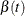.

NOTEST

suppresses the correlation tests.

OUT=SAS-data-set

names the output data set that contains the time-varying coefficients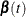, one row per event time. The variables that containhave the same names as the predictors. The data set also contains the transformed event times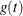.

TRANSFORM=IDENTITY | KM | LOG | RANK

specifies how the failure times should be transformed in the diagnostic plots and correlation tests. You can choose from the following transformations:

IDENTITY

specify the identity transformation,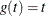.

KM

specifies the complement of the Kaplan-Meier estimate transformation,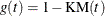.

LOG

specifies the log transformation,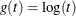.

RANK

specifies the rank transformation,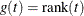.